# Understanding the Half Angle Formulas

The half-angle formulas can be used to reduce the power of a squared trig function.

The half-angle formulas are: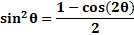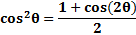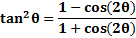Examples: Rewrite each expression to contain only first-degree functions:

1)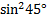We must use the formula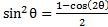to reduce the power. Notice that 45° is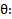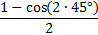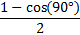We could stop here, but if you remember that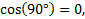we can go further: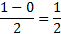2)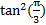We must use the formula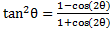to reduce the power. Notice thatis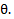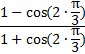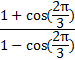3)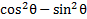We aren't told what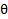equals in this expression, so we will work with it as is. Let's replace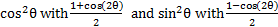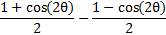And since they have the same denominator we can subtract the numerators: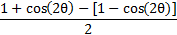Next we'll distribute and combine like terms: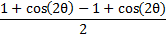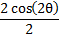And reduce: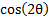Practice: Rewrite each expression to contain only first-degree trig functions

1)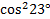2)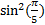3)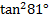4)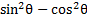5)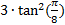Answers:1)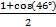2)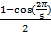3)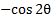4)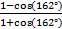5)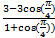Related Links: Math Fractions Factors